# Finding straight lines and circles in log-polar images

## Introduction to log-polar sampling

Log-polar sampling is a spatially-variant image representation in which pixel separation increases linearly with distance from a central point . It provides a way of concentrating computational resources on regions of interest, whilst retaining low-resolution information from a wider field of view. Foveal image representations like this are most useful in the context of active vision systems, where the densely sampled central region can be directed to pick up the most salient information. Human eyes are, very roughly speaking, organised in this way.

 A log-polar grid with 16 rings and 16 wedges superimposed on a 180 x 180 pixel image. Each sample in a 16 x 16 log-polar image would be derived from the grey levels in one segment of this grid.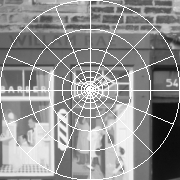The same image sampled on a log-polar grid with 180 rings and 180 wedges, displayed on orthogonal axes, ring number horizontal and wedge number vertical, the origin at the bottom left and top left corners (since the wedge number wraps round). Moving up a column of this image corresponds to moving anticlockwise round a ring in the original image, starting at 3 o'clock. Although log-polar samples are often displayed in this way, the internal representation is no more "distorted" than any other image representation - it is just a different mapping from array indices to image position.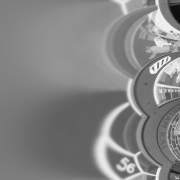The log-polar image above displayed with veridical mapping onto the plane. (Bilinear interpolation was used to display the image.) Note the loss on resolution near the periphery - e.g. the number 54 on the door at the right - compared to the original image.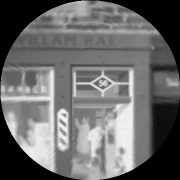In log-polar sampling, pixels are indexed by ring number R and wedge number W, related to ordinary x, y image coordinates by the mapping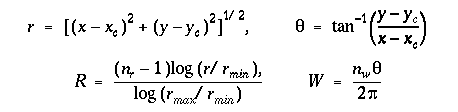where (r, theta) are polar coordinates, (xc, yc) is the position of the centre of the log-polar sampling pattern, nr and nw are the numbers of rings and wedges respectively, and rmin and rmax are the radii of the smallest and largest rings of samples. We also define rho = log r.

A log-polar sampled image is one whose samples are centred on points mapping to integral R and W. In order to keep a pixel's nearest neighbours in orthogonal directions at approximately equal distances from it, the following constraint is needed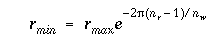## The log-polar straight line

Any straight line, not passing through (xc, yc), can be mapped into any other straight line by a rotation (to make the lines parallel) followed by a uniform expansion with (xc, yc) fixed. This property can be exploited to allow easy detection of lines in log-polar images. Essentially, the log-polar image of a straight line is taken as a template and convolved with the log-polar image under analysis. Peaks in the output correspond to the rotations and expansions that map the template onto matching structures in the image, and so directly give the parameters of detected lines .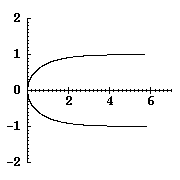The equation of the straight line x=1 in log-polar coordinates is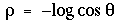and a graph of this equation is shown at the right (rho on the horizonal axis, theta on the vertical). If we convolve the reflected log-polar image of this special straight line with the image of a general line given by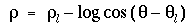then the peak of the convolution output will be at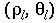.

Since the template is the same size as the image, it is efficient to perform the convolution by multiplication in the Fourier domain. It is possible to find a closed-form expression for the Fourier Transform of the straight line in log-polar space, and also to directly compute the Fourier Transform of spatial derivatives of the mask.

#### Example

 The image at the right has been preprocessed to enhance local contrast and remove very low spatial frequencies.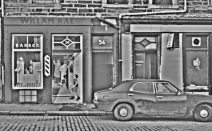The image was then resampled onto a log-polar grid with 128 rings and 256 wedges. This resampled image was convolved with a straight line mask which had been smoothed and differentiated with respect to R. The spatial form of this is shown at the right, though the convolution was carried out using the Fourier transform.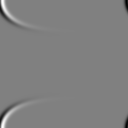Local maxima in the convolution output were found, and ranked by magnitude. A line corresponding to each of these peaks is plotted on the original image at the right.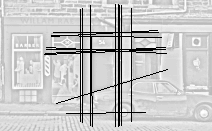The lines found can be truncated to line segments by multiplying the log-polar image pixel by pixel with the spatial form of the convolution mask, having first reflected and translated the mask to make it line up with the detected line. The resulting array contains the values that would have been summed to produce the convolution peak, if the convolution had been carried out in the spatial domain. The size of these values indicates how much each pixel of the image contributed to the peak. We then simply project this array onto the W axis, by summing over all R from 0 to nr-1 for each W, and threshold to find the line limits.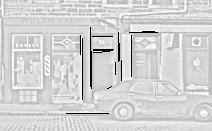An "eye movement" strategy can be used to move the sampling centre across the image to build up the representation of the image structure.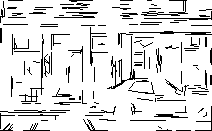## Circle detection in the log-polar image

Since a circle through the sampling centre can be mapped onto any other such circle by a rotation and an expansion, such circles can also be detected by a convolution in log-polar space. Circles passing through the origin are, of course, unlikely to occur by chance. However, if the sampling centre is deliberately placed on a smooth boundary, possibly using the output of log-polar straight line detection, then the circle detected should approximate the osculating circle and the orientation and curvature of the boundary can be estimated.

In fact circles through (xc, yc) can be found for almost no additional computational effort alongside straight lines. Such circles are mirrored straight lines in log-polar coordinates: the equation of the circle centred on (xc+1/2, yc) is rho = log cos theta. Thus the template shown above will match circles if it is simply left-right reflected. A peak in the convolution output gives the point on the circle diametrically opposite to the sampling centre, and hence the circle's centre and radius.

Because of the symmetry between straight lines and circles, a single Fourier transform pair can be used to detect both of them in a single operation.

 A log-polar image displayed on rho, theta axes, with the sampling centre on the upper edge of the car's rear wheel. The car's shadow and the wheel show the reflection symmetry between lines and circles.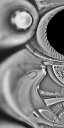The result of convolving the image with a circle mask. Smoothing and differentiation with respect to W were incorporated.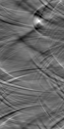The circle corresponding to the maximum of the convolution output (in white), along with four other circles (black) generated the same way at different sampling centres (black dots), each chosen to be close to a curved boundary. Wheel arches are found even though they are not exact or complete circles.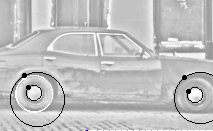## References

 Weiman, C.F.R. and Chaikin, G. (1979) Logarithmic spiral grids for image processing and display. Computer Graphics and Image Processing, 11, 197-226.

 Young, D. (2000) Straight lines and circles in the log-polar image. In M. Mirmehdi & B. Thomas (Eds.), BMVC2000: Proceedings of the 11th British Machine Vision Conference, 11-14 September 2000, The University of Bristol (pp. 426-435). Also at http://www.bmva.ac.uk/bmvc/2000/papers/p43.pdf